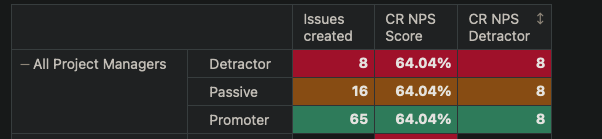# Show measure only to relevant value in Row

Hi,
I created the calculated measure “CR NPS Detractor” that basically filter only the value of the Detractor option, this is my definition:

``````Sum(
{[Customer NPS Rating].[Detractor]},
[Measures].[Issues created]
)
``````Why is it showing the number also for the other options? Is there a way to show it just to the relevant option on the Rows?
Daniel

@Daniel_Luevano
That is because the new measure is ignoring the Customer NPS Rating completely.
It would show same results for any table row

``````CASE WHEN
[Customer NPS Rating].CurrentMember.Name = "Detractor"
THEN
Sum((
[Customer NPS Rating].[Detractor],
[Measures].[Issues created]
))
END
``````

This formula would now be calculated only for one specific row in your report - row where Customer NPS Rating dimension member name is “Detractor”

Martins / eazyBI

Looks good @martins.vanags, but now how can I do to show also the totals on ‘All Customer NPS Ratings’?

@Daniel_Luevano
Try this code

``````CASE WHEN
[Customer NPS Rating].CurrentMember.Name = "Detractor"
THEN
Sum((
[Customer NPS Rating].[Detractor],
[Measures].[Issues created]
))
WHEN
[Customer NPS Rating].CurrentMember.Name = "All Customer NPS Ratings"
THEN
Sum([Measures].[Issues created] )
END
``````

Martins / eazyBI